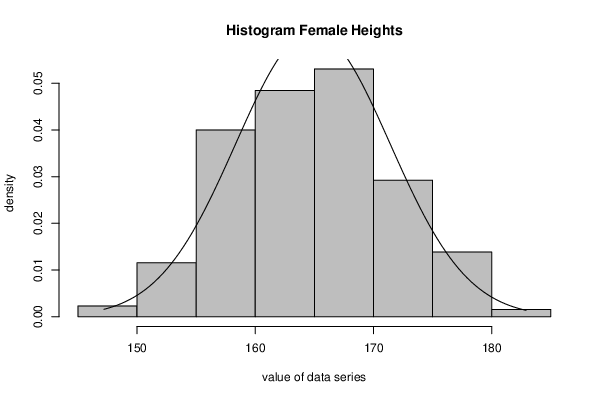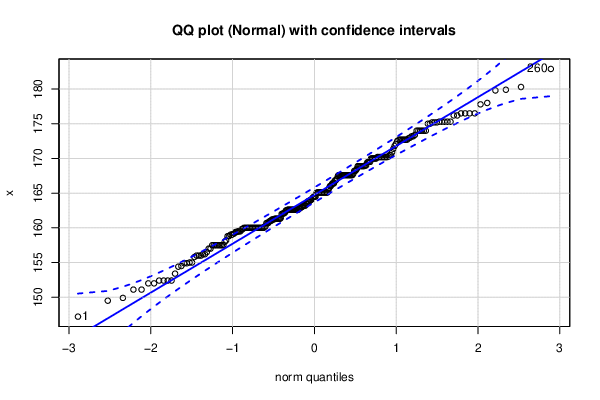Free Statistics

of Irreproducible Research!

Author's title
Author*Unverified author*
R Software Modulerwasp_fitdistrnorm.wasp
Title produced by softwareML Fitting and QQ Plot- Normal Distribution
Date of computationSat, 06 Feb 2021 22:15:44 +0100
Cite this page as followsStatistical Computations at FreeStatistics.org, Office for Research Development and Education, URL https://freestatistics.org/blog/index.php?v=date/2021/Feb/06/t1612646236pxbhaszdud26fvb.htm/, Retrieved Tue, 18 Jan 2022 14:22:31 +0000
Statistical Computations at FreeStatistics.org, Office for Research Development and Education, URL https://freestatistics.org/blog/index.php?pk=319365, Retrieved Tue, 18 Jan 2022 14:22:31 +0000
QR Codes:Original text written by user:
IsPrivate?No (this computation is public)
User-defined keywords
Estimated Impact33
Family? (F = Feedback message, R = changed R code, M = changed R Module, P = changed Parameters, D = changed Data)
-       [ML Fitting and QQ Plot- Normal Distribution] [] [2021-02-06 21:15:44] [d41d8cd98f00b204e9800998ecf8427e] [Current]
Feedback Forum

Post a new message
Dataseries X:
147.2
149.5
149.9
151.1
151.1
152.0
152.0
152.4
152.4
152.4
152.4
153.4
154.4
154.5
154.9
154.9
155.0
155.0
155.8
156.0
156.0
156.0
156.2
156.2
156.5
157.0
157.0
157.5
157.5
157.5
157.5
157.5
157.5
157.5
157.5
158.0
158.2
158.8
158.8
159.0
159.0
159.1
159.2
159.4
159.4
159.5
159.5
159.5
159.8
159.8
160.0
160.0
160.0
160.0
160.0
160.0
160.0
160.0
160.0
160.0
160.0
160.0
160.0
160.0
160.0
160.0
160.0
160.0
160.0
160.0
160.2
160.2
160.7
160.7
160.7
160.9
161.0
161.0
161.2
161.2
161.2
161.3
161.3
161.3
161.3
161.3
161.3
161.3
161.4
162.0
162.0
162.1
162.1
162.2
162.5
162.5
162.6
162.6
162.6
162.6
162.6
162.6
162.6
162.6
162.6
162.6
162.6
162.6
162.6
162.6
162.8
162.8
162.9
163.0
163.0
163.2
163.2
163.2
163.2
163.5
163.5
163.8
163.8
163.8
164.0
164.0
164.1
164.3
164.4
164.5
164.5
164.5
165.0
165.1
165.1
165.1
165.1
165.1
165.1
165.1
165.1
165.1
165.1
165.1
165.1
165.1
165.1
165.5
165.7
166.0
166.0
166.2
166.4
166.4
166.4
166.8
166.8
167.0
167.1
167.5
167.6
167.6
167.6
167.6
167.6
167.6
167.6
167.6
167.6
167.6
167.6
167.6
167.6
167.6
167.6
167.6
167.6
167.8
168.2
168.2
168.3
168.5
168.9
168.9
168.9
168.9
168.9
168.9
168.9
168.9
169.0
169.0
169.4
169.5
169.5
169.5
170.0
170.0
170.0
170.0
170.0
170.0
170.2
170.2
170.2
170.2
170.2
170.2
170.2
170.2
170.2
170.2
170.3
170.5
170.5
170.9
171.4
171.8
172.1
172.5
172.7
172.7
172.7
172.7
172.7
172.7
172.7
172.9
173.0
173.2
173.2
173.4
174.0
174.0
174.0
174.0
174.0
174.0
175.0
175.0
175.2
175.2
175.2
175.3
175.3
175.3
175.3
175.3
176.2
176.2
176.5
176.5
176.5
176.5
177.8
178.0
179.8
179.9
180.3
182.9

 Summary of computational transaction Raw Input view raw input (R code) Raw Output view raw output of R engine Computing time 1 seconds R Server Big Analytics Cloud Computing Center

\begin{tabular}{lllllllll}
\hline
Summary of computational transaction \tabularnewline
Raw Input view raw input (R code)  \tabularnewline
Raw Outputview raw output of R engine  \tabularnewline
Computing time1 seconds \tabularnewline
R ServerBig Analytics Cloud Computing Center \tabularnewline
\hline
\end{tabular}
%Source: https://freestatistics.org/blog/index.php?pk=319365&T=0

[TABLE]
[ROW]
 Summary of computational transaction[/C][/ROW] [ROW] Raw Input[/C] view raw input (R code) [/C][/ROW] [ROW] Raw Output[/C] view raw output of R engine [/C][/ROW] [ROW] Computing time[/C] 1 seconds[/C][/ROW] [ROW] R Server[/C] Big Analytics Cloud Computing Center[/C][/ROW] [/TABLE] Source: https://freestatistics.org/blog/index.php?pk=319365&T=0

Globally Unique Identifier (entire table): ba.freestatistics.org/blog/index.php?pk=319365&T=0

As an alternative you can also use a QR Code:

The GUIDs for individual cells are displayed in the table below:

 Summary of computational transaction Raw Input view raw input (R code) Raw Output view raw output of R engine Computing time 1 seconds R Server Big Analytics Cloud Computing Center

 Parameter Estimated Value Standard Deviation mean 164.872307692308 0.40509770564045 standard deviation 6.53200423223537 0.286447334701474

\begin{tabular}{lllllllll}
\hline
Parameter & Estimated Value & Standard Deviation \tabularnewline
mean & 164.872307692308 & 0.40509770564045 \tabularnewline
standard deviation & 6.53200423223537 & 0.286447334701474 \tabularnewline
\hline
\end{tabular}
%Source: https://freestatistics.org/blog/index.php?pk=319365&T=1

[TABLE]
[ROW][C]Parameter[/C][C]Estimated Value[/C][C]Standard Deviation[/C][/ROW]
[ROW][C]mean[/C][C]164.872307692308[/C][C]0.40509770564045[/C][/ROW]
[ROW][C]standard deviation[/C][C]6.53200423223537[/C][C]0.286447334701474[/C][/ROW]
[/TABLE]
Source: https://freestatistics.org/blog/index.php?pk=319365&T=1

Globally Unique Identifier (entire table): ba.freestatistics.org/blog/index.php?pk=319365&T=1

As an alternative you can also use a QR Code:

The GUIDs for individual cells are displayed in the table below:

 Parameter Estimated Value Standard Deviation mean 164.872307692308 0.40509770564045 standard deviation 6.53200423223537 0.286447334701474PNG link Postscript link PDF linkPNG link Postscript link PDF link

Parameters (Session):
par1 = 8 ; par2 = 0 ;
Parameters (R input):
par1 = 8 ; par2 = 0 ;
R code (references can be found in the software module):
par2 <- '0'
par1 <- '8'
library(MASS)
library(car)
par1 <- as.numeric(par1)
if (par2 == '0') par2 = 'Sturges' else par2 <- as.numeric(par2)
x <- na.omit(x)
x <- as.ts(x) #otherwise the fitdistr function does not work properly
r <- fitdistr(x,'normal')
print(r)
bitmap(file='test1.png')
myhist<-hist(x,col=par1,breaks=par2,main=main,ylab=ylab,xlab=xlab,freq=F)
curve(1/(r$estimate*sqrt(2*pi))*exp(-1/2*((x-r$estimate)/r$estimate)^2),min(x),max(x),add=T) dev.off() bitmap(file='test3.png') qqPlot(x,dist='norm',main='QQ plot (Normal) with confidence intervals') grid() dev.off() load(file='createtable') a<-table.start() a<-table.row.start(a) a<-table.element(a,'Parameter',1,TRUE) a<-table.element(a,'Estimated Value',1,TRUE) a<-table.element(a,'Standard Deviation',1,TRUE) a<-table.row.end(a) a<-table.row.start(a) a<-table.element(a,'mean',header=TRUE) a<-table.element(a,r$estimate)
a<-table.element(a,r$sd) a<-table.row.end(a) a<-table.row.start(a) a<-table.element(a,'standard deviation',header=TRUE) a<-table.element(a,r$estimate)
a<-table.element(a,r\$sd)
a<-table.row.end(a)
a<-table.end(a)
table.save(a,file='mytable.tab')